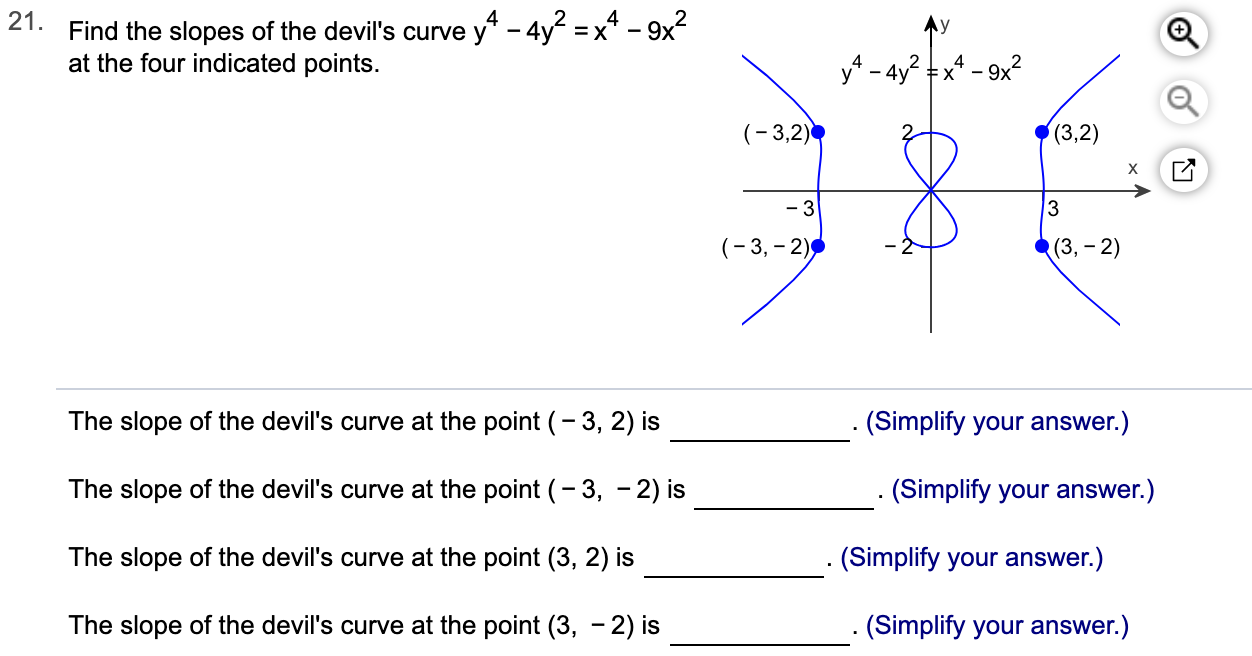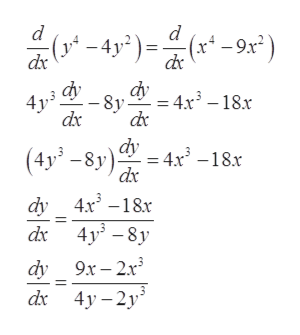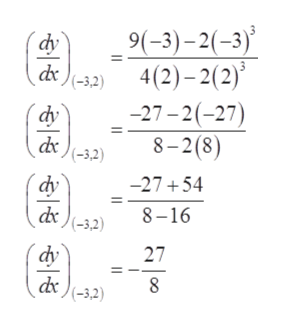y* - 4y2 =x4 -9x221. Find the slopes of the devil's curveat the four indicated points.y4- 4y2 x4-9x2(3,2)(3,2)х-33(3,-2)(-3, 2)The slope of the devil's curve at the point (-3, 2) is(Simplify your answer.)The slope of the devil's curve at the point (-3, -2) is(Simplify your answer.)The slope of the devil's curve at the point (3, 2) is(Simplify your answer.)The slope of the devil's curve at the point (3, - 2) is. (Simplify your answer.)

Question

Can you help me with this problem step by step?help_outlineImage Transcriptionclosey* - 4y2 =x4 -9x2 21. Find the slopes of the devil's curve at the four indicated points. y4- 4y2 x4-9x2 (3,2) (3,2) х -3 3 (3,-2) (-3, 2) The slope of the devil's curve at the point (-3, 2) is (Simplify your answer.) The slope of the devil's curve at the point (-3, -2) is (Simplify your answer.) The slope of the devil's curve at the point (3, 2) is (Simplify your answer.) The slope of the devil's curve at the point (3, - 2) is . (Simplify your answer.) fullscreen
Step 1

Consider the given function.

Step 2

Since, the derivative of the curve gives the slope of the given curve.

So, differentiate the above function with respect to xhelp_outlineImage Transcriptionclosed d (x -9x) -4y2 dx 8y 4x3 -18.x 4y dx dy 4x18.x (4y-8y) dy 4x -18x d 4y8y dy 9x-2r3 dx 4y-2y fullscreen
Step 3

Now, the slope of the given func...help_outlineImage Transcriptionclose9(-3)-2(-3)' 4 (2)-2(2) -27-2(-27) 8-2(8) dy d )(-3,2) dy (-3,2) dy dx )(-32) -27+54 8-16 27 dy 8 dx )(-3,2) fullscreen

Want to see the full answer?

See Solution

Want to see this answer and more?

Our solutions are written by experts, many with advanced degrees, and available 24/7

See Solution
Tagged in

Derivative